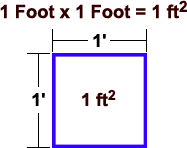Directions: Enter any combination of feet and/or inches into the calculator below. You can use this triangle square footage calculator to calculate siding square footage in gables or any other triangle square feet you might need (like: roofing, carpet, etc.) You can also use this tool to convert feet to inches, convert inches to feet, calculate triangle square inches.# Square Footage Calculator Triangle

(Height x Length) ÷ 2 = Triangle Square Footage)
(Triangle Square Footage = Triangle Square Feet = Tri SqFt = Tri Sq.Ft. = tri sq.ft. = tri ft2)
(

## Height

feet     inches
X

## Length

feet     inches) ÷ 2

## Directions:

Use the calculator above to calculate a triangle's square footage.
You can enter feet only, inches only or a combination of the two.
*Square Footage is also known as (a.k.a) square feet, square ft, SqFt, Sq.Ft., sq.ft., ft2 & ft2
**This Calculator also calculates triangle square inches, the triangle's total Height in feet, total Height in inches, total length in feet and total length in inches.## What is a Square Foot?

A "square foot" is a unit of area measurement equal to a square measuring one foot on each side. 1 Square Foot = 0.0929 square meters. Abbrev.: ft2, sq.ft, SqFt.

Articles